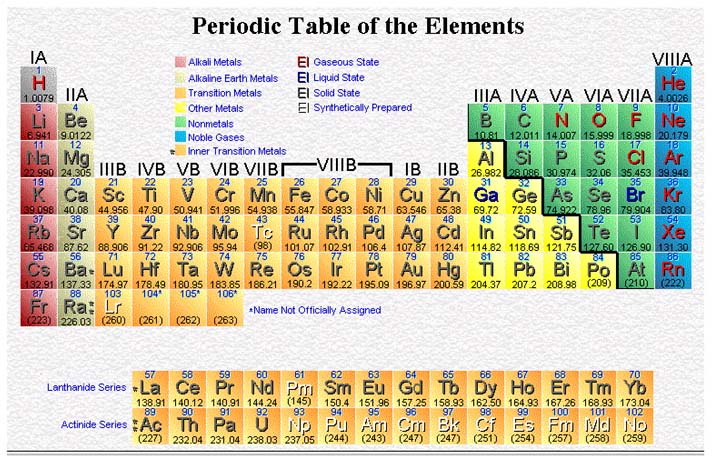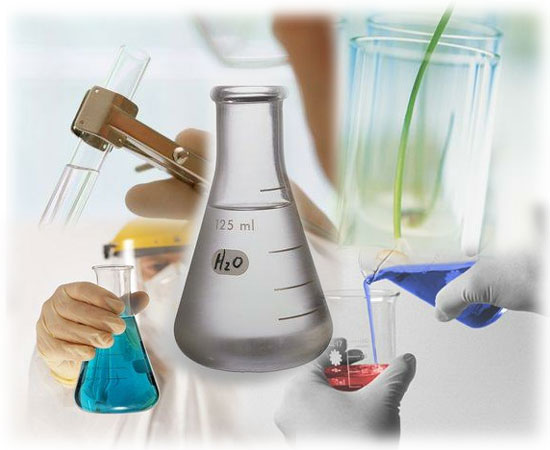CHEMISTRY Pre-AP Unit III: Atomic Structure and Nuclear Chemistry Chapters 4, 25Goal: The student will gain an understanding of atomic theory and nuclear chemistry using the Bohr-Rutherford Model of the atom.

Objectives: The student should be able to:

1. Define an atom.

2. Describe the atomic models of Democritus and Aristotle.

3. List and interpret the assumptions of Dalton’s Atomic Theory and indicate which assumptions have been modified based on more current knowledge.

4. Identify the information gained about the atom when given descriptions of early experiments.

5. Describe the Thompson model of the atom.

6. Describe the Bohr-Rutherford model of the atom, including what portion of the atom is empty space and where most of the mass is located.

7. Locate the three fundamental particles in the atom; indicate the relative mass and charge of these particles.

8. Indicate what the atomic number and mass number of an atom represent.

9. Calculate the mass number when given the number of protons and neutrons.

10. Indicate which isotope is used as the standard for atomic mass.

11. Write the nuclear symbol for an atom.

12. Determine the number of protons, neutrons, and electrons when given the atomic number and mass number.

13. Calculate average atomic mass from relative abundance.

14. Define isotope and identify which nuclides are isotopes of the same element when given the atomic number and mass number of these nuclides.

15. Describe the three most common radioactive emissions in terms of relative charge, relative mass and origin.

16. Apply three tests for relative stability of nuclides.

17. Complete transmutation equations involving alpha, beta, and positron decay, and k-capture.

18. Solve problems involving radioactive decay rates.

19. Compare the processes of fission and fusion and indicate which method is currently employed in nuclear power plants.

20. Identify the role and composition of each of the following parts of a typical nuclear reactor: fuel elements, control rods, moderator and coolant.

21. Explain how energy from a nuclear reactor core is utilized to produce electricity.

23. Describe the optimum conditions for fusion.

24. Describe how Carbon-14 dating is used to determine the age of fossils and other ancient artifacts.

25. Define the following terms:

Reactor core Transmutation

Fissile isotope Plasma

Critical mass Thermal (slow) neutron

Half-life Magnetic bottle

26. Identify several applications of radioisotopes in research and medicine.

27. Describe several methods used to detect and measure radiation.

28. Describe some of the damaging effects of radiation on biological systems.

Activities:

Sep 13: Discuss Unit II Test, Begin Lecture: Democritus and Aristotle, Dalton’s Model, Crooke’s Tube, Thompson

Sep 14: Finish Lecture: Democritus and Aristotle, Dalton’s Model, Crooke’s Tube, Thompson

Sep 15: Lecture: Millikan, Later Atomic Models, Goldstein, Becquerel, Curie

Sep 19: Lecture: How Atoms Differ, Lab: Conservation of Mass

Sep 20: Lecture: Stability of Nuclides, Transmutations, Nuclear reactions

Sep 21: Lecture: Fission and Fusion, Applications and Effects of Nuclear Reactions

Sep 22: Review for test on Chapters 4 and 25

Sep 25: Unit III Test

Homework:

Sep 13: Conservation of Mass Prelab: Read Chapter 4

Sep 14: Chapter 4 Study Guide

Sep 15: Work on Conservation of Mass Lab Report, Due Sep 18

Sep 18: Conservation of Mass Prelab

Sep 19: Work on Conservation of Mass Lab Report, Due Sep 26

Sep 20: Chapter 25 Study Guide

Sep 21: Study for test